# Game Hacks: Finding Objects using Template Matching

Can you use image processing techniques to find objects?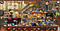Image from Big Home Hidden Objects

Are your eyes tired of looking for hidden objects in games? Do you want an easy way to do it? Friends and family, may I introduce my algorithm to ease your worries. We will use template matching in finding these objects.

What is template matching?

Template matching is an image processing technique of finding the template image (the image that we want to find) from a source image (the bigger image where we need to search for the image). The intuition behind this is to compare the pixel values of the…

# Sudoku Solver using Image Processing and Neural Networks

Can Neural Networks and Image Processing be able to solve sudoku puzzles?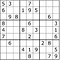Image from Wikipedia

I love solving sudoku puzzles. After reading articles in the local newspaper, I’m solving the sudoku and crossword puzzle. It is a good brain exercise to start the morning. After learning some lessons on image processing, I just thought, can I use what I learned to automatically solve these from scanned images? My teammates and I came up with a simple algorithm to solve this. Many thanks to Tal, Lyon, and Aids for helping me creating this.

Overall Process

The process of making this are the following:

1. Binarization…

# Extracting Data from Photos using Image Processing Techniques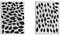Original Image from Gino Borja, AIM

Hello everyone. In this post, we will extract data using image processing techniques. We have two images of leaves here as our example, and the goal is to process the images for machine learning classification. We will be using python libraries. Let’s start.

Overall Process

In this post, we will show how to extract data for both neural networks and traditional machine learning approaches. The overall process consists of the following steps:

1. Binarization
2. Extract Images using Connected Components
3. Extract Region Properties DataFrame

Binarization

Binarization involves changing the image from a multitone image into a two-tone image (black and white). If…

# Introduction to Image Processing — Histogram Manipulation using Skimage

Hello, welcome again to another blog post for the application of skimage. We will try to adjust the image by adjusting their Cumulative Distrubtion Function (CDF) of their intensities. We will again import the required python libraries.

`import numpy as npimport matplotlib.pyplot as pltimport pandas as pdfrom skimage.io import imshow, imreadfrom skimage.color import rgb2grayfrom skimage import img_as_ubyte, img_as_floatfrom skimage.exposure import histogram, cumulative_distribution`

Let’s check the first time image below.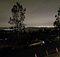Image from Author

The image is dark, and we want it to look a little bit brighter. Let’s check first the histogram value of the image in grayscale space…

# Introduction to Image Processing — Color Overcast Adjustment using Python

In this article, we will focus on how to handle images with color overcast. These kinds of images usually happen when you have either an underexposure or overexposure. Let’s get started by uploading the needed libraries.

`import numpy as npimport matplotlib.pyplot as pltimport pandas as pdfrom skimage.io import imshow, imreadfrom skimage.util import img_as_ubyte`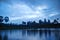Image Taken by Author

The image has mostly bluish in color and overcast. Let's investigate the RGB spectrum of the image.

`image_overcast = imread(“siemreap.jpg”)rgb_list = [‘Reds’,’Greens’,’Blues’]fig, ax = plt.subplots(1, 3, figsize=(15,5), sharey = True)for i in range(3): ax[i].imshow(image_overcast[:,:,i], cmap = rgb_list[i]) ax[i].set_title(rgb_list[i], …`

# Introduction to Image Processing — Color Spaces

Python is a fun programming language for exploring around with color spaces in images. I hope this story will give you some ideas on the basic types of color spaces. In this story, we will use Yuyuko as our sample.Image: Touhouwiki.net

Let’s get started first with some important libraries in Python.

`import numpy as npimport matplotlib.pyplot as pltfrom skimage import img_as_uintfrom skimage.color import rgb2hsvfrom skimage.io import imshow, imreadfrom skimage.color import rgb2grayfrom skimage.color import rgb2lab`
1. RGB Model

The RGB Model is a color model that can produce various colors by “additive” combinations of the primary colors…

## Kris Tabong

Learning more about Image Processing using Python